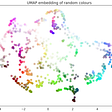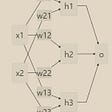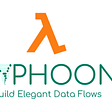# Trained a Machine Learning Model in Pure SQL

## Because recursive CTEs can “iterate”, it’s possible to implement machine learning model training in pure SQL — without Python at all

In the article Deep Neural Network implemented in pure SQL over BigQuery, the author claimed to use pure SQL to implement a deep neural network model. But after I opened his repo, I found that he used Python to implement iterative training, which was not truly pure SQL.

In this post, I’ll share how I trained a machine learning model in pure SQL on TiDB, an open-source distributed SQL database. Major steps included:

1. Choosing the Iris dataset
2. Choosing the softmax logistic regression model for training
3. Writing an SQL statement to implement model inference
4. Training the model

In my test, I trained a softmax logistic regression model. During the test, I found that TiDB did not allow subqueries and aggregate functions in recursive common table expressions (CTEs). By modifying TiDB’s code, I bypassed the limitations and successfully trained a model and got a 98% accuracy rate on the Iris dataset.

# Why I chose TiDB for implementing the machine learning model

TiDB 5.1 has introduced many new features, including common table expressions (CTEs) of the ANSI SQL 99 standard. We can use a CTE as a statement for a temporary view to decouple a complex SQL statement and develop code more efficiently. What’s more, a recursive CTE can refer to itself. This is important for improving SQL functionality. Moreover, CTEs and window functions make SQL a Turing-complete language.

Because recursive CTEs can “iterate,” I wanted to try and see whether I could use pure SQL to implement machine learning model training and inference on TiDB.

# The Iris dataset

I chose the Iris dataset at scikit-learn. This data set contains 150 records of 3 types, each with 50 records. Each record has 4 features: sepal length (sl), sepal width (sw), petal length (pl), and petal width (pw). We can use these features to predict whether an iris belongs to iris-setosa, iris-versicolour, or iris-virginica.

After I downloaded the data in CSV format, I imported it into TiDB.

# Softmax logistic regression

I chose a simple machine learning model: softmax logistic regression for multi-class classification.

In softmax regression, the probability of classifying x into category y is:

The cost function is:

# Model inference

I wrote an SQL statement to implement inference. Based on the model and data defined above, the input data x had five dimensions (sl, sw, pl, pw, and a constant 1.0). The output used one-hot encoding.

There were 15 parameters: 3 types * 5 dimensions.

I initialized the input data to 0.1, 0.2, 0.3. I used different numbers for the convenience of demonstration. Initializing all of them to 0.1 is OK.

Next, I wrote a SQL statement to count the result accuracy for data inference. For better understanding, I used pseudo code to describe this process:

In the code above, I calculated elements in each row of the data. To make a sample’s inference:

1. I got the EXP of the weighted vectors.
2. I got the softmax value.
3. I chose the largest of p0, p1, and p2 as 1; I set the rest to 0.

If the inference result of a sample is consistent with its original classification, it is a correct prediction. Then, I summed the correct numbers of all samples to get the final accuracy rate.

The following code shows the implementation of the SQL statement. I joined each row of data with a weight (only one row of data), calculated the inference result of each row, and summed the correct numbers of samples:

The SQL statement above almost implements the calculation process of the pseudo code step by step. I got the result:

Next, I’ll learn the model parameters.

# Model training

Note: To simplify the problem, I did not consider the “training set” and “validation set” issues, and I used all the data only for training.

I wrote pseudo code and then wrote a SQL statement based on it:

Because I manually expanded the sum and w vectors, this code looked a little cumbersome.

Then, I started to write SQL training. First, I wrote an SQL statement with only one iteration.

I set the learning rate and the number of samples:

The code iterated once:

The result was model parameters after one iteration:

The following is the core part. I used recursive CTEs for iterative training:

The core idea is that the input of each iteration was the result of the previous iteration, and I added an incremental iteration variable to control the number of iterations. The general framework was:

Next, I combined the SQL statement of an iteration with this iteration framework. To improve the calculation accuracy, I added type conversions to the intermediate results:

There were two differences between this code block and the code block with one iteration above. In this code block:

• After `data join weight`, I added `where iter <@num_iterations` to control the number of iterations and the `iter + 1 as iter` column to the output.
• I added `having count(*)> 0` to prevent the aggregation from outputting data when there was no input data at the end. This error might cause the iteration to fail to end.

The result was:

This showed that recursive CTEs did not allow subqueries in the recursive part. But I could merge all the subqueries above. After I merged them manually, I got this:

It showed that aggregate functions were not allowed.

Then, I decided to change TiDB’s implementation.

According to the introduction in the proposal, the implementation of recursive CTEs followed the basic execution framework of TiDB. After I consulted Wenjun Huang, an R&D at PingCAP, I learned that there were two reasons why subqueries and aggregate functions were not allowed:

• MySQL did not allow them.
• If allowed, there would be a lot of complicated corner cases.

But I just wanted to test the features. I deleted the check of subqueries and aggregate functions temporarily in diff.

I executed the code again:

It was a success! I got the parameters after 1,000 iterations.

Next, I used the new parameters to recalculate the correct rate:

This time, the accuracy rate reached 98%.

# Conclusion

By using recursive CTEs in TiDB 5.1, I successfully used pure SQL to train a softmax logistic regression model on TiDB.

During the test, I found that TiDB’s recursive CTEs did not allow subqueries and aggregate functions, so I modified TiDB’s code to bypass these limitations. Finally, I successfully trained a model and obtained a 98% accuracy rate on the Iris dataset.

My work also uncovered a couple of ideas I’d like your thoughts on. If these topics interest you, please join the TiDB community on Slack and discuss them with me.

• After I did some tests, I found that neither PostgreSQL nor MySQL supported aggregate functions in recursive CTEs. There might be corner cases that were difficult to handle.
• In this test, I manually expanded all dimensions of the vectors. In fact, I also wrote an implementation that did not need to expand all dimensions. For example, the schema of the data table was (idx, dim, value), but in this implementation, the weight table needed to be joined twice. This means that it needed to be accessed twice in the CTE. This also required modification of the implementation of TiDB executor. Therefore, I didn’t talk about it in this article. But in fact, this implementation was more general, which could handle models with more dimensions, for example, the MNIST dataset.

--

--

--

## More from Geek Culture

A new tech publication by Start it up (https://medium.com/swlh).

## Artificial Intelligence in Mechanical Engineering## Interpreting Accuracy, Precision, Recall, and F1 Score Metrics## IBM Watson Assistant Is Focusing On Enhancing Irrelevance Detection In New Releases## Mini NLP Cypher | Mini Year Review## UMAP clearly explained## How To Use Microsoft LUIS With Bot Framework Composer## How Text Recognition Works (Part 3)## [NLP] Ensembling in PyTorch## PingCAP

PingCAP is the team behind TiDB, an open-source MySQL compatible NewSQL database. Official website: https://pingcap.com/ GitHub: https://github.com/pingcap

## Impress your friends! Make a serverless bot that sends daily jokes to a Telegram Group## The Way of the Cache## HOLA Optimization: A Lightweight Hyperparameter Optimization Software Package## Fast Multivalue Look-ups For Huge Data Sets